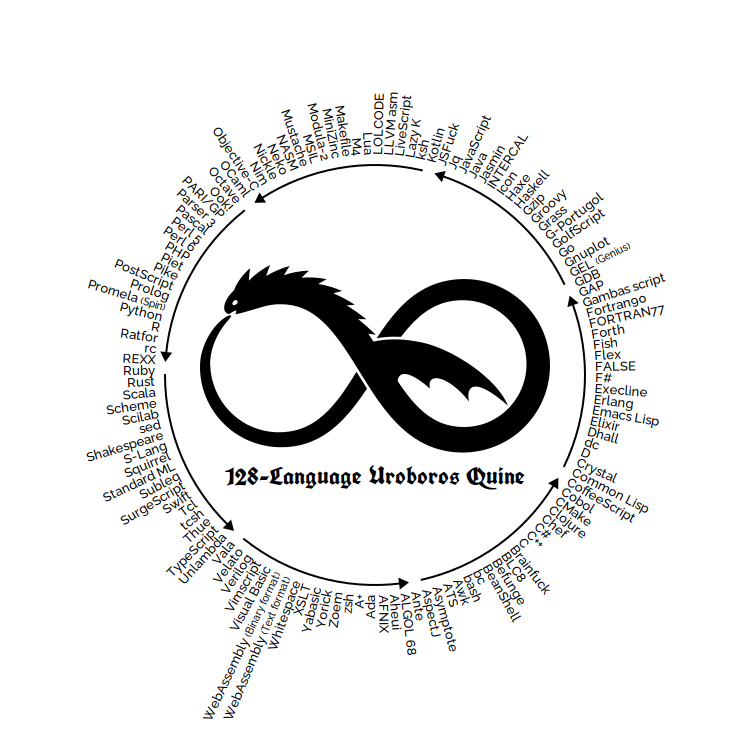#### 海选文章:12 导向自由的律令# 又名: 迎接另一个新天地

… …

• 我说:”想当年我们也是雄姿英发的在做梦,怎么少了今天这份从容和自由的体会?”
• 他很平淡的说:”当年我们都在池里呀. “

2013-11-20   HaiDoc

#### D码点评:12 自食# 语言的quine接力

``````q a b c=putStrLn \$ b ++ [toEnum 10,'q','('] ++ show b ++ [','] ++ show
c ++ [','] ++ show a ++ [')']main=q "q a b c=putStrLn \$ b ++
[toEnum 10,'q','('] ++ show b ++ [','] ++ show c ++ [','] ++ show a ++
[')']" "def q(a,b,c):print b+chr(10)+'q('+repr(b)+','+repr(c)+','+repr(
a)+')'" "def e(x) return 34.chr+x+34.chr end;def q(a,b,c) print b+
10.chr+'main=q '+e(b)+' '+e(c)+' '+e(a)+' '+10.chr end"
``````

2013-11-19   gDgcoDe

# [傳家]中國人的生活智慧«传家»简体中文版编辑报告

2013-11-18   GdgBook